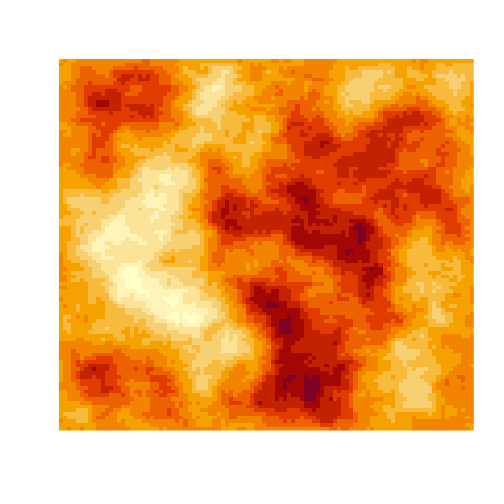# Stefan Siegert

## Sampling the 2d random walk

The RW2D is a 2-dimensional random field $$u_{i,j}$$ characterised by the intrinsic auto-regression $u_{i,j} = \frac14 (u_{i-1,j} + u_{i+1,j} + u_{i,j-1} + u_{i,j+1}) + \epsilon_{i,j}$ where $$\epsilon_{i,j} \sim N(0,\tau^{-1})$$ is independent Gaussian noise with precision $$\tau$$. The RW2D is widely used as a spatial prior in Bayesian hierarchical modelling.

Sampling realisations of the RW2D is not completely straightforward, since $$u_{i,j}$$ depends on its neighbors, which in turn also depend on their neighbors. There is no natural starting point. Time series models like AR(1) or the random walk have a well-specified "forward direction" which makes sampling straightforward. Sampling the RW2D on the other hand needs a bit more work.

Let the $$n \times n$$ matrix $$U$$ denote a realisation of the RW2D random field. Define the tri-diagonal $$n \times n$$ matrix $$D$$ as $D = \frac14 \begin{pmatrix} 2 & -1 & \\ -1 & \ddots & \ddots \\ & \ddots \end{pmatrix}.$

Then the matrix $$E$$ defined by $E = DU + UD$ is a $$n \times n$$ matrix of iid Normal random variables with mean zero and precision $$\tau$$.

This is a Sylvester equation so it can be rewritten in vectorised form as $vec(E) = (1_n \otimes D + D \otimes 1_n) vec(U)$ where $$1_n$$ is the $$n$$-dimensional identity matrix, $$\otimes$$ is the Kronecker product, and the vectorisation operator $$vec(\cdot)$$ transforms a matrix into a vector by column-stacking.

The vectorised equation suggests a way to simulate an RW2D random field $$U$$ by first generating a vector $$e$$ of $$n^2$$ iid Normal variates, and multiplying it by the inverse of the 2d differencing matrix, i.e. $u = (1_n \otimes D + D \otimes 1_n)^{-1} e.$ Recasting the $$n^2$$ vector $$u$$ back into a $$n \times n$$ matrix $$U$$ yields a realisation of an RW2D.

In R, this is done as follows:

library(Matrix)
set.seed(123)
n = 100
In = Diagonal(n)
Dn = 0.25 * bandSparse(n=n, k=0:1, symmetric=TRUE,
diagonals=list(rep(2, n), rep(-1, n-1)))
DD = kronecker(In, Dn) + kronecker(Dn, In)
uu = solve(DD, rnorm(n^2))
U = matrix(uu, n, n)
image(U, axes=FALSE)